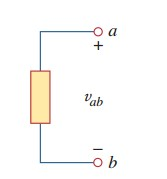omG8HI9LSKFW6lhCj8prTs0Z6lUhdOT9Jhi1Sf4m

# Electric Voltage Definition

From the explanation before, in order to move the electron from one point to another, we need some work or energy transfer.

## Electrical Voltage

This work is happened by electromotive force (emf) externally, and also represented the battery illustration.

Before moving on, make sure to read what is dc circuit first.

This emf can be called by voltage or potential difference. In an electric circuit, voltage vab in between points a and b is needed to move a unit charge from a to b as energy or work, in the mathematic equation:(1)

where :
w = work (joules = J)
q  = charge (coulombs = C)

The voltage vab is measured in volts (V), to respect the physicist inventor, Alessandro Antonio Volta (1745 - 1827), the inventor of the first voltaic battery. From Equation.(1):

1 volt =  1 joule/coulomb = 1 newton-meter/coulomb
Hence,
Voltage or potential difference is required energy to move a unit charge through an elements, measured in volts (V).Figure 1. Potential difference
An easy understanding of voltage can be seen in Figure.(1). The voltage across an element connected to points a and b. The symbol plus (+) and minus(-) represent the current flow direction or voltage polarity.

There are two ways to treat vab : (1) potential at point a is higher than point b, or (2) potential at point a with respect to point b is vab. Logically in general :
For better understanding, here we review Figure.(2). Two representations of the same voltage at 9 V has different polarity based on the polarity symbol. In Figure.(2a), point a is +9V above point b, while Figure.(2b), point b is -9V above point a

From this perspective, we can conclude that in Figure.(2a) there is 9V voltage drop from a to b, and in Figure.(2b) there is 9V voltage rise from b to a. In other words, the voltage drop in Figure.(2a) is equivalent to voltage rise in Figure.(2b).Figure 2. Voltage polarity
Like current, voltage also has its form depending on its value in a period of time. The constant value of voltage is known as dc voltage (represented by V) and the dynamic one is ac voltage (represented by v).

Along with electrical current, these two can be used for the calculation of electric power.
Have you understood what is electric voltage?Don't forget to share and subscribe!Happy learning!
Reference:  Fundamentals of electric circuits by Charles K. Alexander and Matthew N. O. Sadiku

Untuk Bahasa Indonesia baca Tegangan Listrik.
Related Posts
SHARE